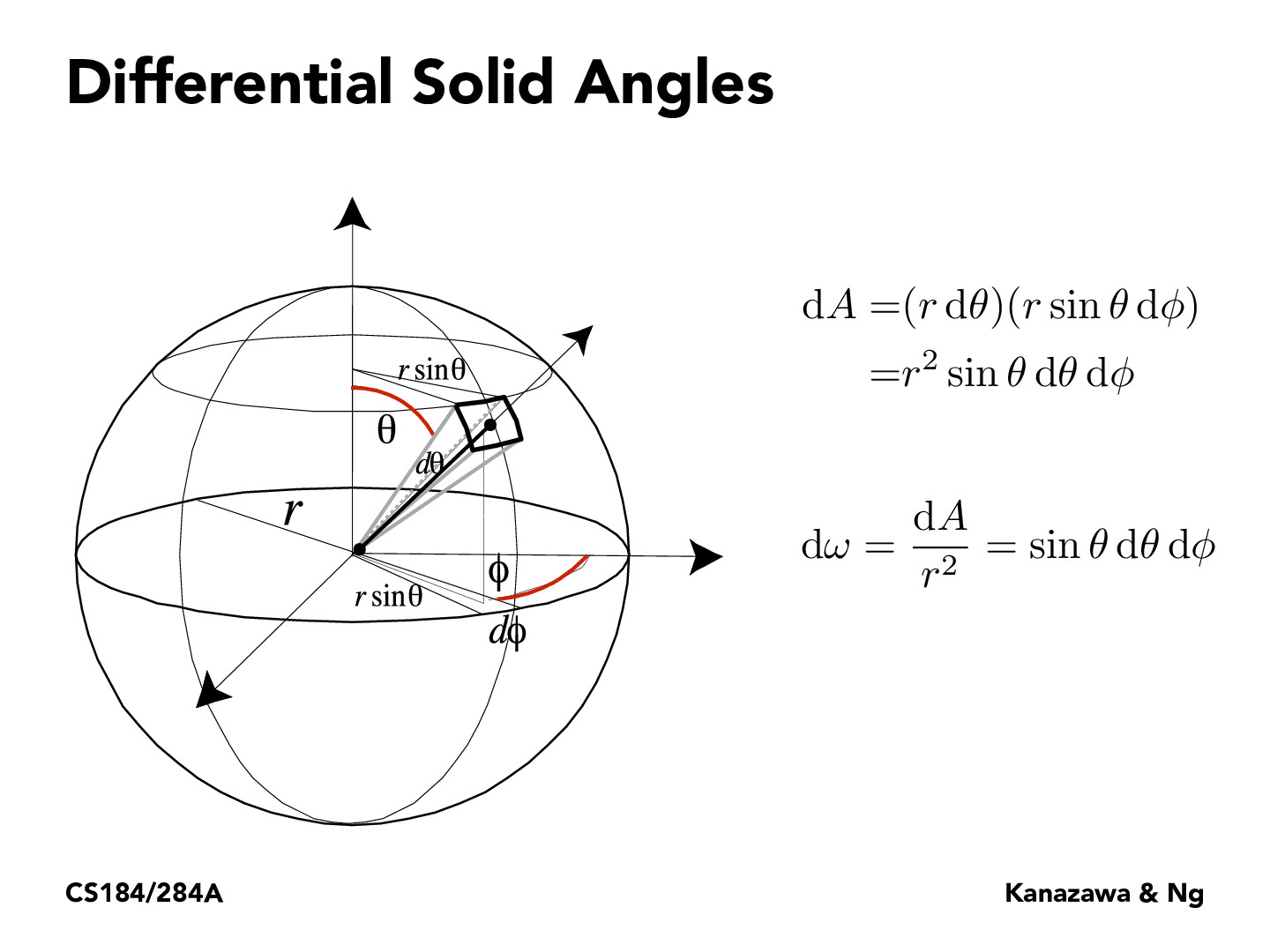Lecture 11: Radiometry & Photometry (15)philippe-eecs

I'm confused on how dA was derived exactly here. $$rd\theta$$ is the arc length of the first portion, but is $$r sin(\theta)d\phi$$ the width in this case? Is there another more intuitive way to derive this?briana-jin-zhang

The idea is that when dtheta is really small, you can view the cut surface as a rectangle. You are right, r sin theta dphi is the width. The way you can derive r sin theta dphi is that there is going to be an upside down right triangle with base r sin theta as the top of the triangle. This is the length/radius from the center now in a sense. Then you multiply by dphi since we are finding arc length.

You must be enrolled in the course to comment